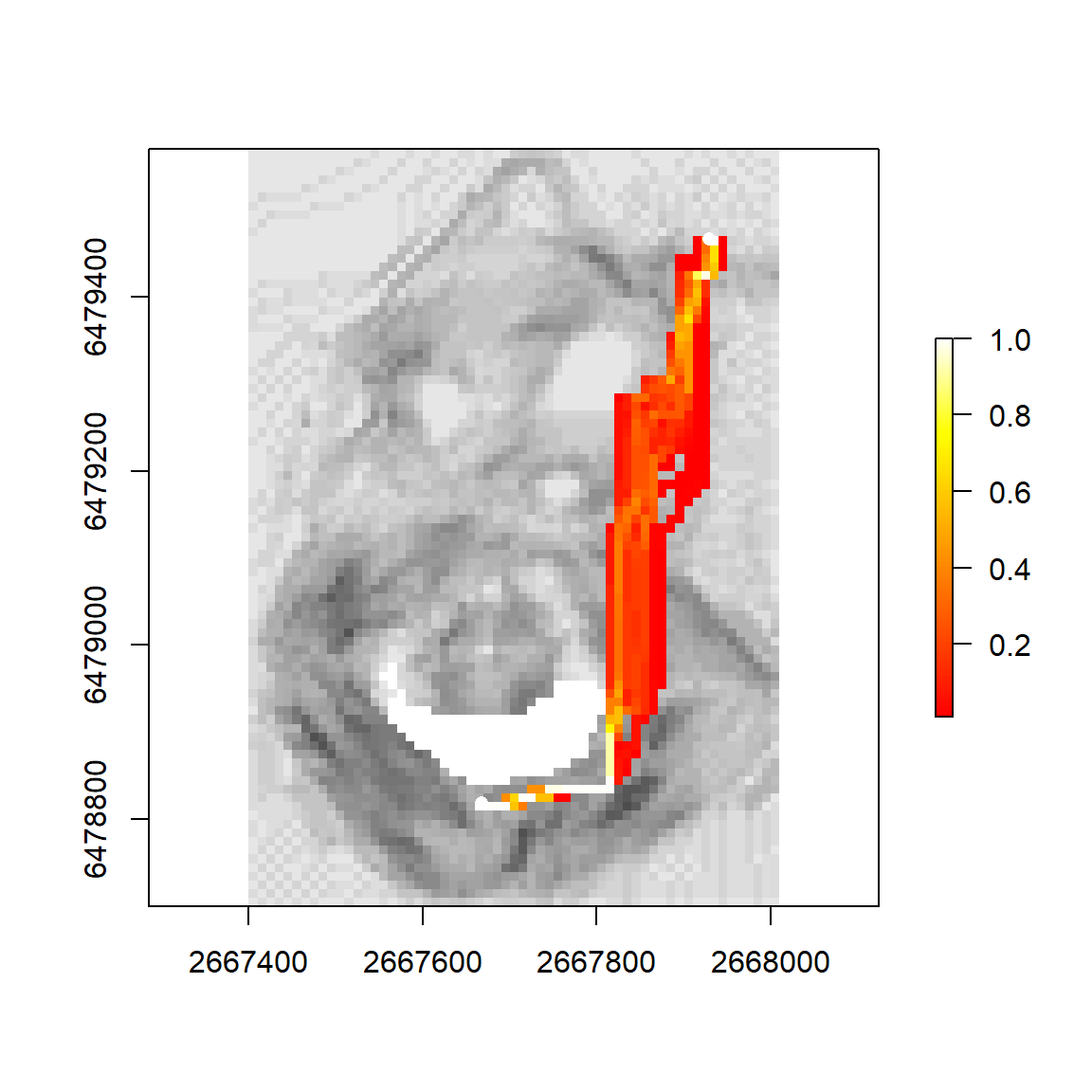# 1. Introducing leastcostpath

This vignette describes leastcostpath, a package written for use in the R environment (R Core Team, 2018) and built on classes and functions provided in the R package gdistance (Van Etten, 2017). leastcostpath provides the functionality to calculate Least Cost Paths (LCPs) using numerous cost functions (time- and energy-based) that approximate the difficulty of moving across a landscapee. Furthermore, additional cost surfaces can be incorporated into the analysis via create_barrier_cs() or create_feature_cs().

Secondly, leastcostpath provides the functionality to calculate Stochastic Least Cost Paths (Pinto and Keitt, 2009); and Probabilistic Least Cost Paths (Lewis, 2020).

Thirdly, leastcostpath provides functionality to calculate movement potential within a landscape through the implementation of From-Everywhere-to-Everywhere (White and Barber, 2012); Cumulative Cost Paths (Verhagen 2013), and Least Cost Path calculation within specified distance bands (Llobera, 2015).

Lastly, leastcostpath provides the functionality to validate the accuracy of the computed Least Cost Path relative to another path via validate_lcp() (Goodchild and Hunter, 1997) and PDI_validation() (Jan et al. 1999).

# 2. using leastcostpath

## 2.1 Setup

library(rgdal)
library(rgeos)
library(sp)
library(raster)
library(gdistance)
library(leastcostpath)
dem <- raster::raster(system.file('external/maungawhau.grd', package = 'gdistance'))

dem_extent <- as(raster::extent(dem), 'SpatialPolygons')

raster::crs(dem_extent) <- raster::crs(dem)

raster::plot(dem)
raster::plot(dem_extent, add = T, border = "red")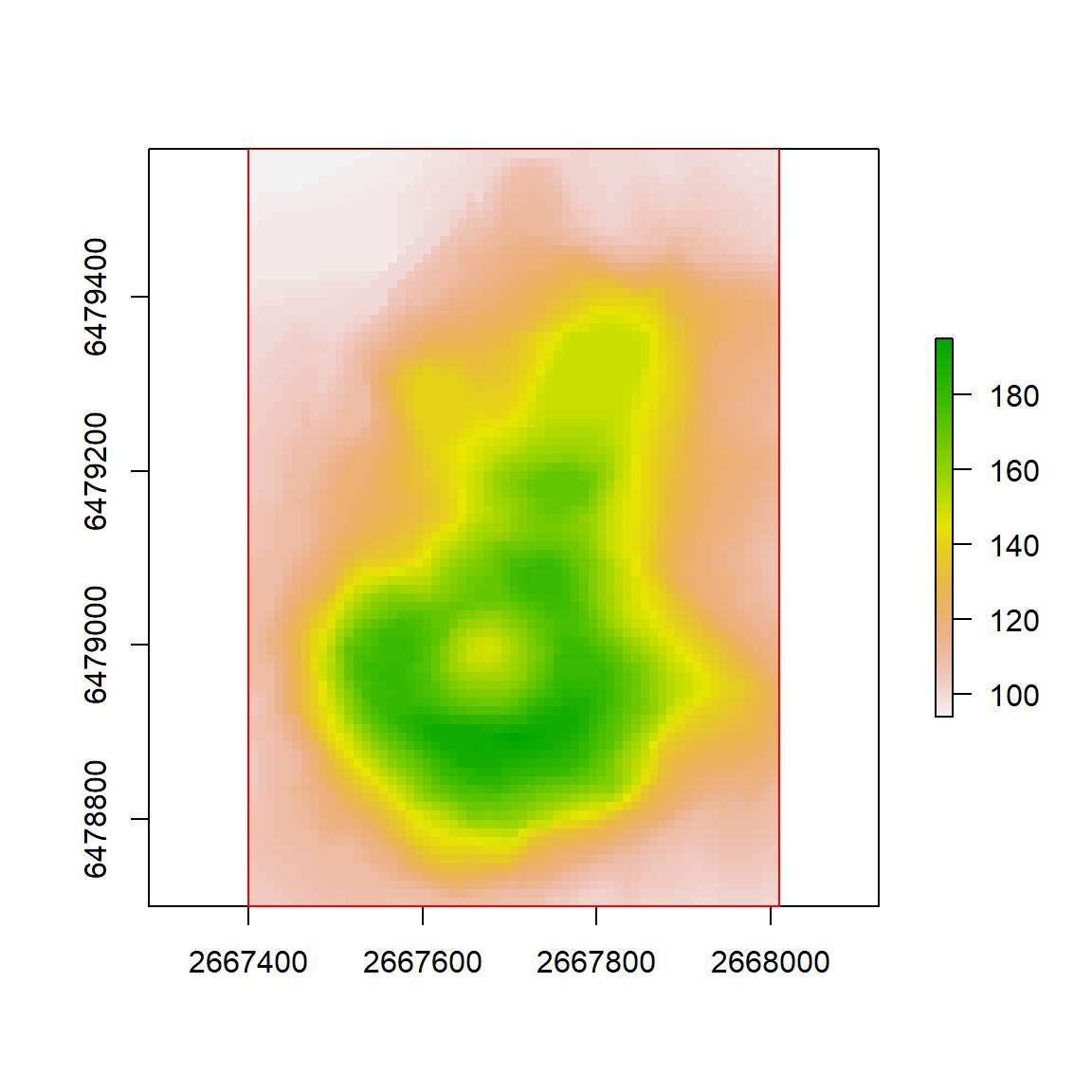## 2.2 Creating Slope-based Cost Surfaces

# cost functions currently implemented within leastcostpath

cfs <- c("tobler", "tobler offpath", "irmischer-clarke male", "irmischer-clarke offpath male", "irmischer-clarke female", "irmischer-clarke offpath female",
"modified tobler", "wheeled transport", "herzog", "llobera-sluckin", "campbell 2019")

# neighbours can be 4, 8, 16, 32, or 48. 8 used for illustration purposes, but greater the number the better the cost surface / LCP approximates reality.
neigh <- 4

slope_cs <- leastcostpath::create_slope_cs(dem = dem, cost_function = "tobler", neighbours = neigh)

plot(raster(slope_cs), col = grey.colors(100))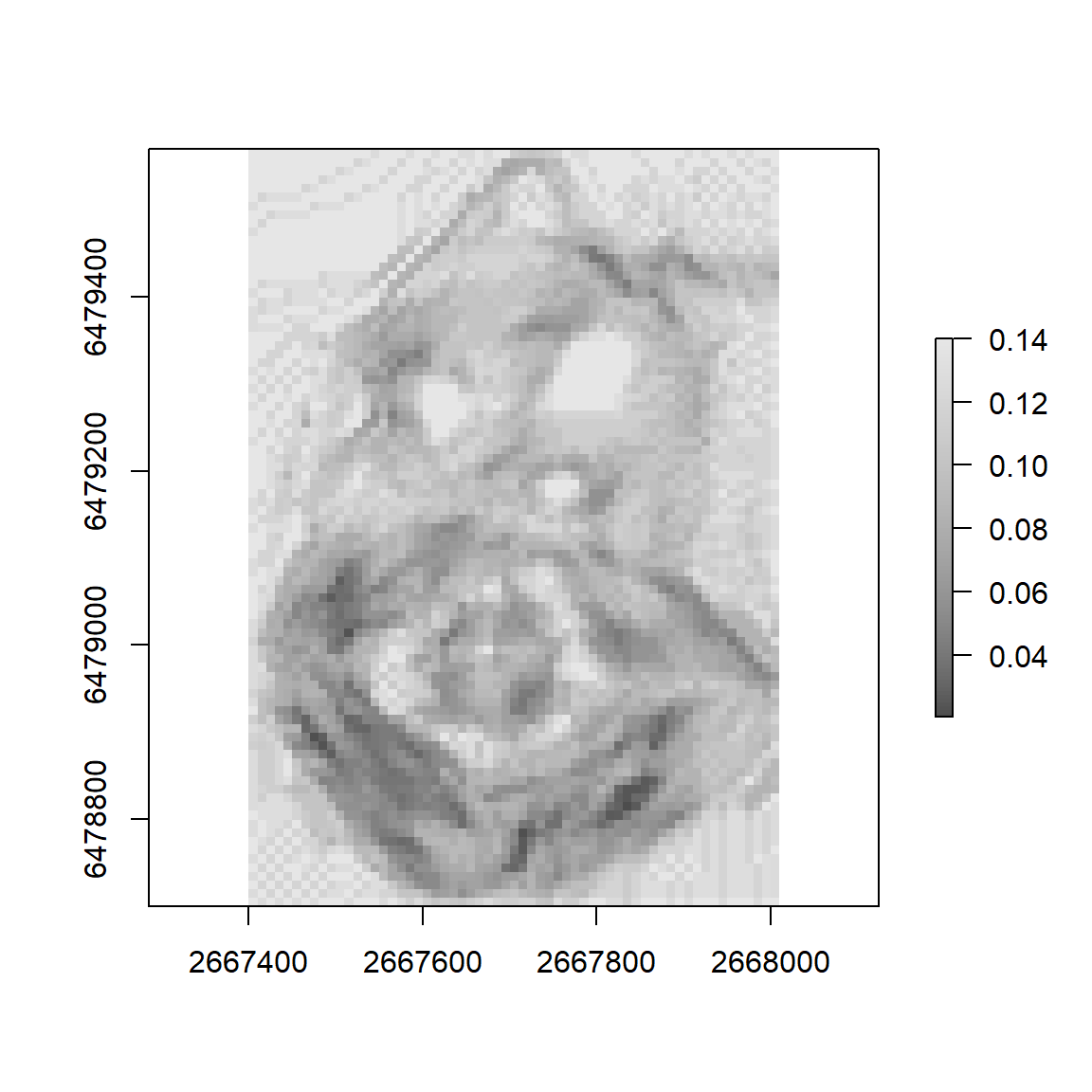## 2.3 Creating and Incorporating Barriers within Cost Surfaces

# create barrier of altitude values above 180

altitude <- dem > 180
# convert value 0 to NA - this ensures that the areas below 180 are not viewed as barriers. That is, if the raster value is NA then create_barrier_cs() will assume that the cell is background and will therefore assign the background argument value
altitude[altitude == 0] <- NA

# the values NOT NA will be assigned the field argument value. If 0 (default) then movement within the area will be completely inhibited.
altitude_cs <- leastcostpath::create_barrier_cs(raster = altitude, barrier = altitude, neighbours = neigh, field = 0, background = 1)

# multiplying the two cost surfaces ensures that barriers continue to completely inhibit movement (i.e. slope_cs values * 0 = 0)
slope_altitude_cs <- slope_cs * altitude_cs

plot(raster(slope_altitude_cs), col = grey.colors(100))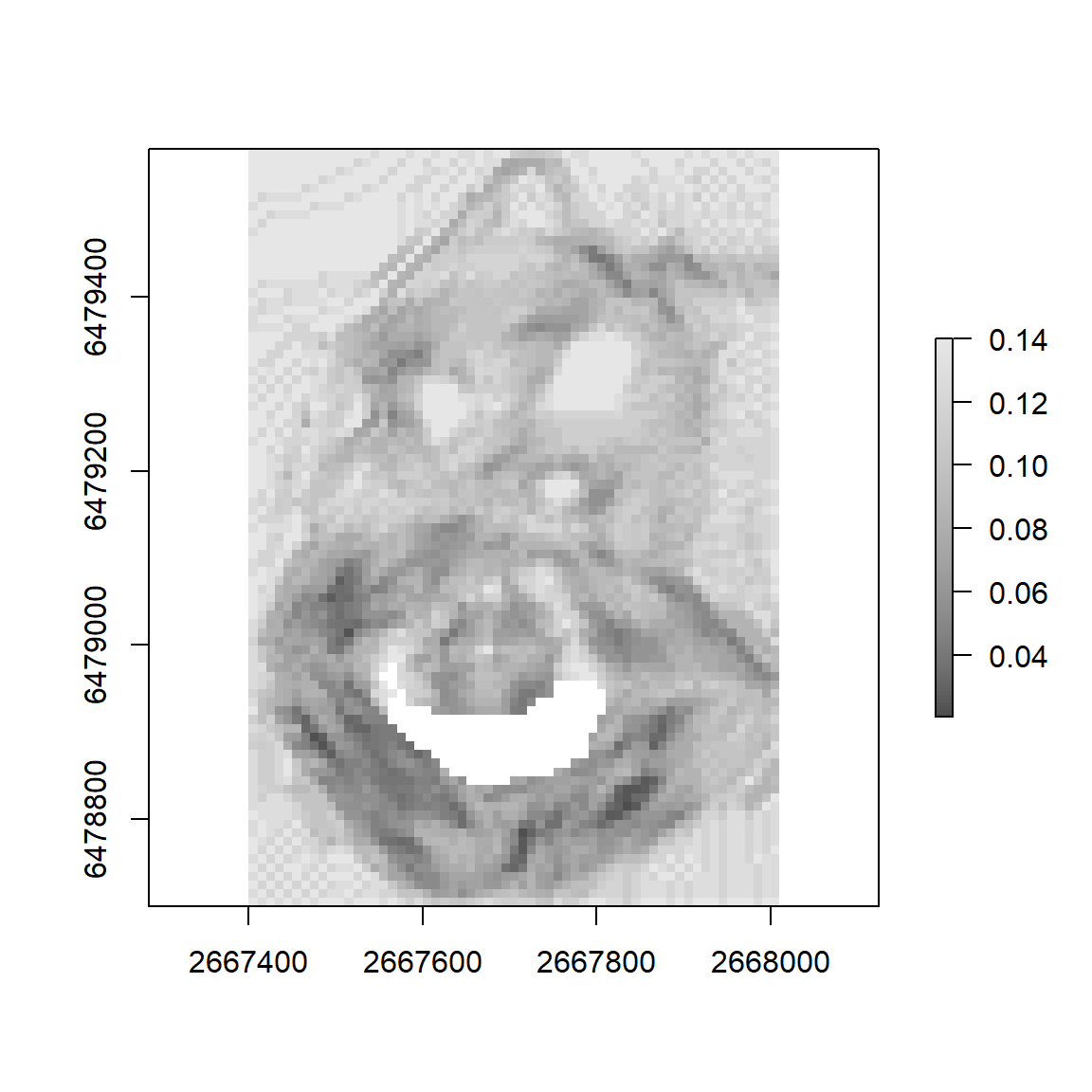## 2.3 Calculating Least Cost Paths

A <- sp::SpatialPoints(cbind(2667930, 6479466))
B <- sp::SpatialPoints(cbind(2667668, 6478818))

# if cost function is anisotropic (e.g. Tobler's Hiking Function) then LCP from A-to-B and B-to-A may be different. To create LCP in both directions set the directional argument to FALSE (default)
lcp <- leastcostpath::create_lcp(cost_surface = slope_altitude_cs, origin = A, destination = B, directional = FALSE)

plot(raster(slope_altitude_cs), col = grey.colors(100))
plot(A, add = T, col = "black")
plot(B, add = T, col = "black")
# LCP from A-to-B
plot(lcp[1,], add = T, col = "red")
# LCP from B-to-A
plot(lcp[2,], add = T, col = "blue")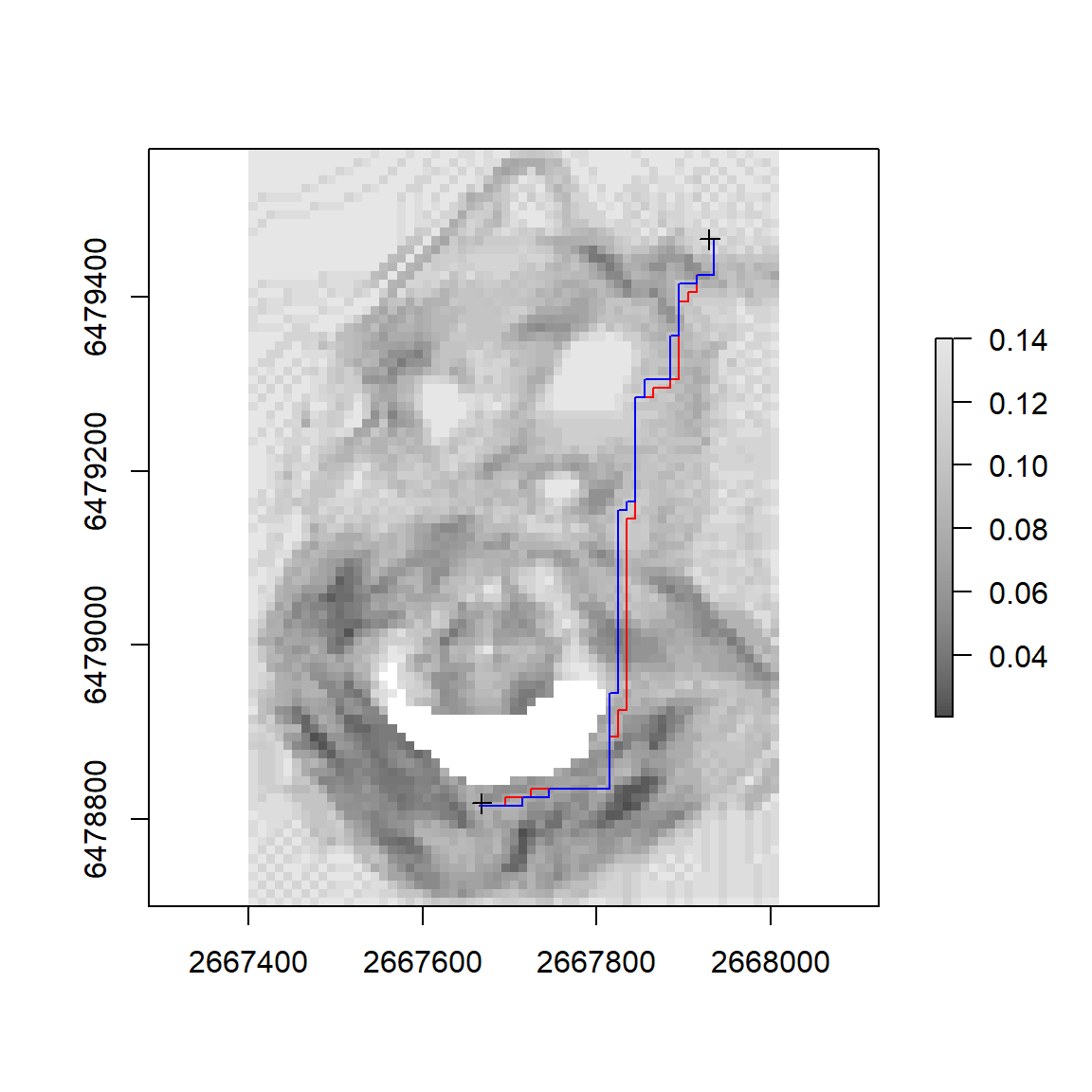## 2.4 Calculating Cost Corridors

# calculates accumulated cost surface from A and from B. These are then averaged. rescale argument TRUE (not default) rescales the accumulated cost surface to 1
cc <- leastcostpath::create_cost_corridor(cost_surface = slope_altitude_cs, origin = A, destination = B, rescale = TRUE)

plot(cc, col = heat.colors(100))
plot(A, add = T, col = "white", pch = 16)
plot(B, add = T, col = "white", pch = 16)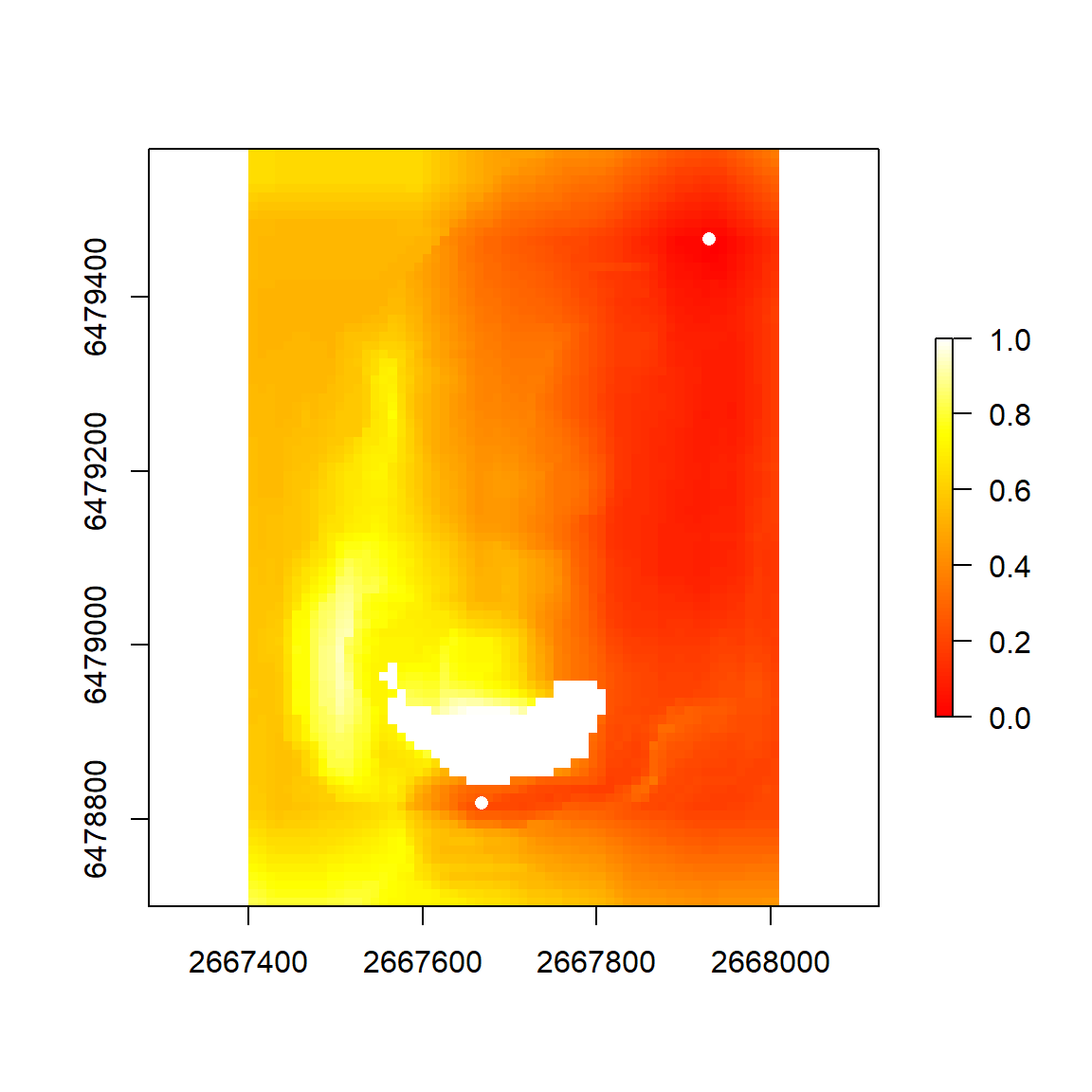## 2.5 Calculating From-Everywhere-To-Everywhere Least Cost Paths

random_locs <- sp::spsample(x = dem_extent, n = 25, type = 'random')

fete_lcp <- leastcostpath::create_FETE_lcps(cost_surface = slope_altitude_cs, locations = random_locs, cost_distance = FALSE, parallel = FALSE)

plot(raster(slope_altitude_cs), col = grey.colors(100))
plot(fete_lcp, add = T, col = "red")
plot(random_locs, add = T, col = "black", pch = 16)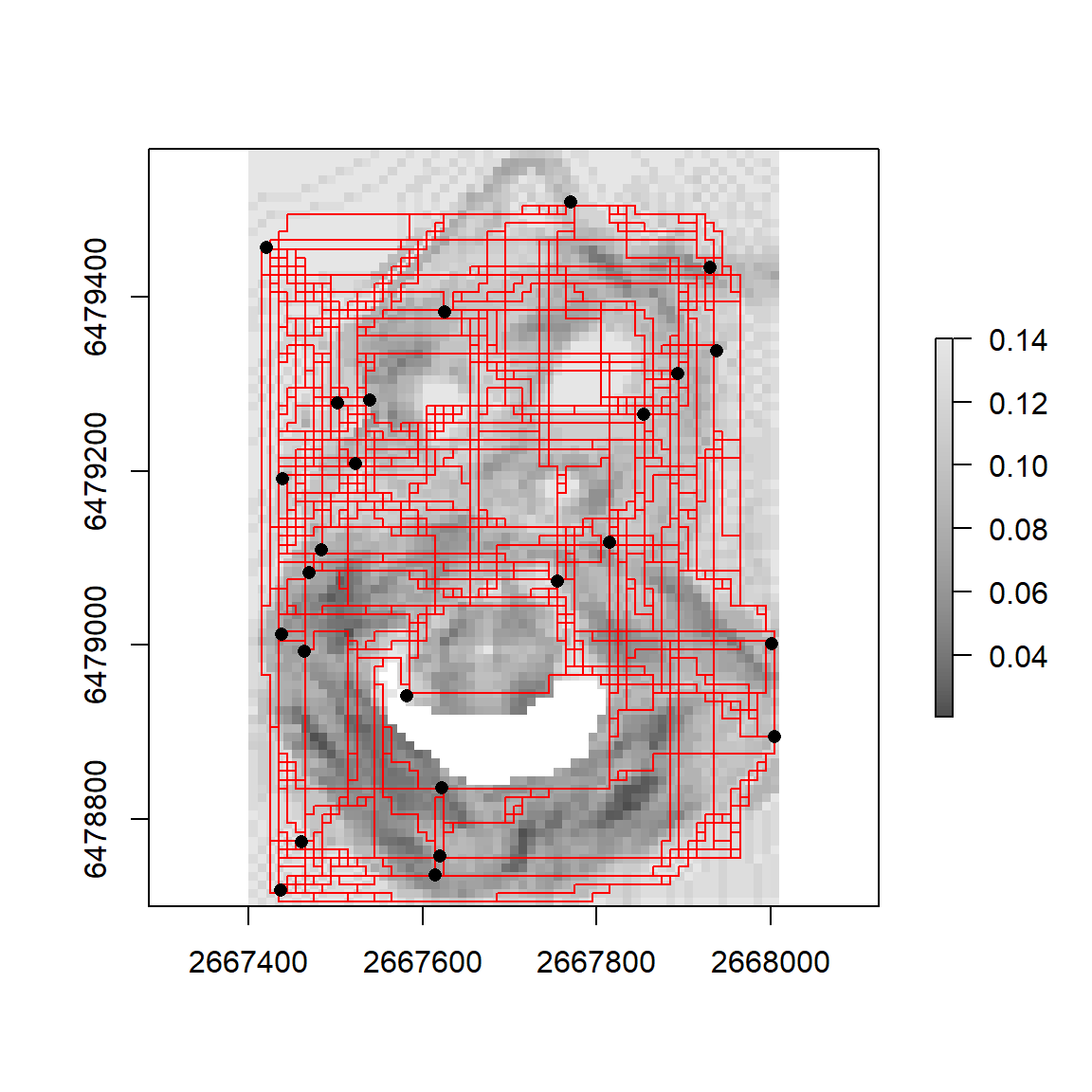## 2.6 Least Cost Path Density

# rasterises the LCPs and calculates the number of times an LCP crosses a raster cell. If rescale argument TRUE (not default) then LCP density scaled to 1. If rasterize_as_points argument TRUE (default) then LCP vertices points are rasterized. This is quicker than rasterizing lines (rasterize_as_lines argument FALSE) but will contain 'gaps' where no LCP vertex point is present
fete_lcp_density <- leastcostpath::create_lcp_density(lcps = fete_lcp, raster = dem, rescale = TRUE, rasterize_as_points = TRUE)

fete_lcp_density[fete_lcp_density == 0] <- NA

plot(fete_lcp_density, col = heat.colors(100))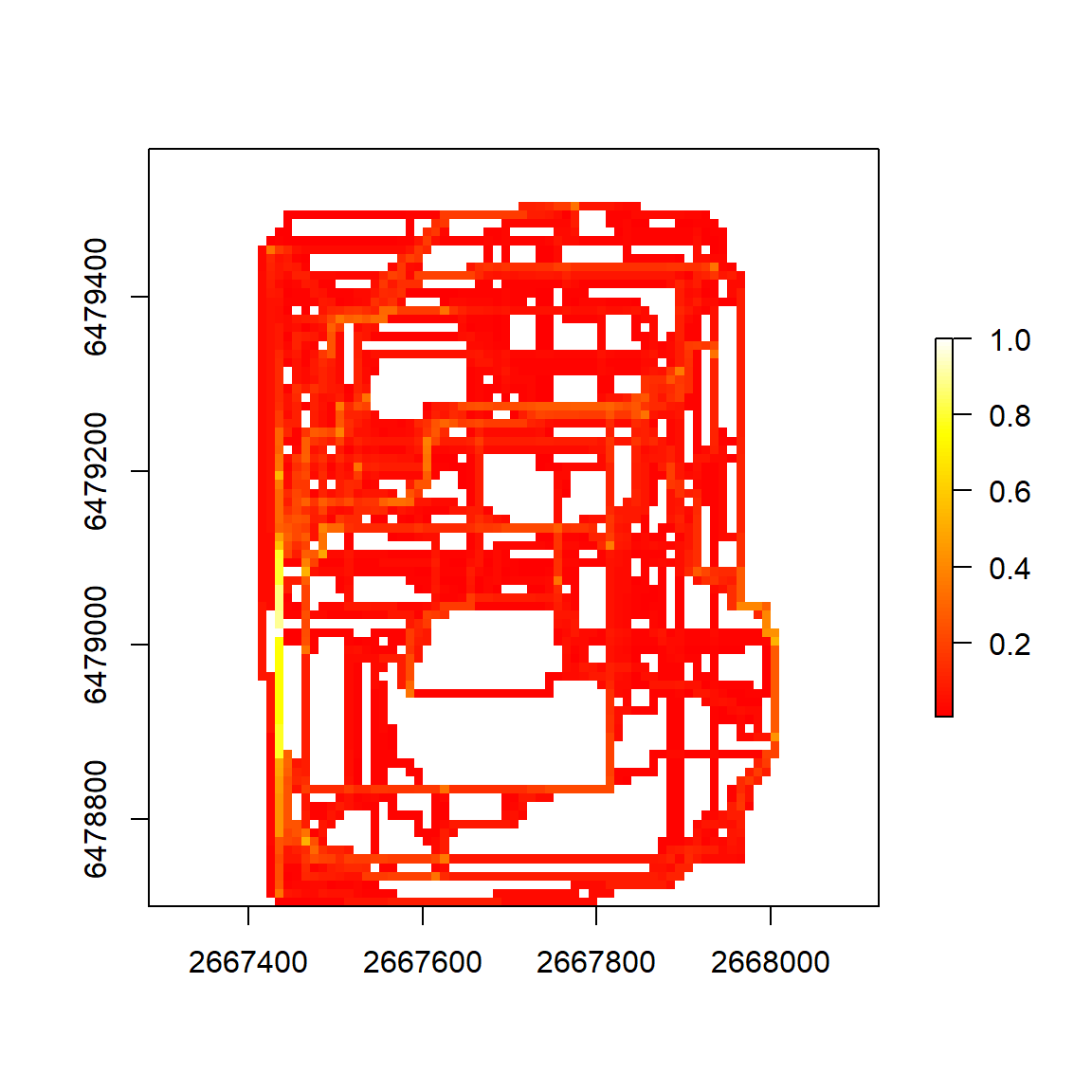## 2.7 Probabilistic Least Cost Paths

# least cost paths are calculated using input data that contains errors. The most notable is the Digital Elevation Model (DEM) which is used to model movement difficulty within the landscape. By incorporating DEM error (in this case vertical error), the error is propagated throughout the LCP modelling process. This results in the input data better approximating the real world, with the LCP being more reliable as a result

# for illustration purposes, we will assume that the vertical error (root-mean-square-error) is +/- 0.5m.
RMSE <- 0.5
n <- 100
lcps <- list()

for (i in 1:n) {

print(i)

lcps[[i]] <- leastcostpath::create_lcp(cost_surface = leastcostpath::create_slope_cs(dem = leastcostpath::add_dem_error(dem = dem, rmse = RMSE, type = "autocorrelated"), cost_function = "tobler", neighbours = neigh) * altitude_cs, origin = A, destination = B, directional = FALSE)

}
##  1
##  2
##  3
##  4
##  5
##  6
##  7
##  8
##  9
##  10
##  11
##  12
##  13
##  14
##  15
##  16
##  17
##  18
##  19
##  20
##  21
##  22
##  23
##  24
##  25
##  26
##  27
##  28
##  29
##  30
##  31
##  32
##  33
##  34
##  35
##  36
##  37
##  38
##  39
##  40
##  41
##  42
##  43
##  44
##  45
##  46
##  47
##  48
##  49
##  50
##  51
##  52
##  53
##  54
##  55
##  56
##  57
##  58
##  59
##  60
##  61
##  62
##  63
##  64
##  65
##  66
##  67
##  68
##  69
##  70
##  71
##  72
##  73
##  74
##  75
##  76
##  77
##  78
##  79
##  80
##  81
##  82
##  83
##  84
##  85
##  86
##  87
##  88
##  89
##  90
##  91
##  92
##  93
##  94
##  95
##  96
##  97
##  98
##  99
##  100
lcps <- do.call(rbind, lcps)

lcps_A_B <- leastcostpath::create_lcp_density(lcps = lcps[lcps$direction == "A to B",], raster = dem, rescale = TRUE, rasterize_as_points = TRUE) lcps_A_B[lcps_A_B == 0] <- NA lcps_A_B <- (lcps_A_B/n) lcps_B_A <- leastcostpath::create_lcp_density(lcps = lcps[lcps$direction == "B to A",], raster = dem, rescale = FALSE, rasterize_as_points = TRUE)

lcps_B_A[lcps_B_A == 0] <- NA

lcps_B_A <- (lcps_B_A/n)

plot(raster(slope_altitude_cs), legend = FALSE, col = grey.colors(100))
plot(lcps_A_B, add = T, col = heat.colors(100))
plot(A, add = T, col = "white", pch = 16)
plot(B, add = T, col = "white", pch = 16)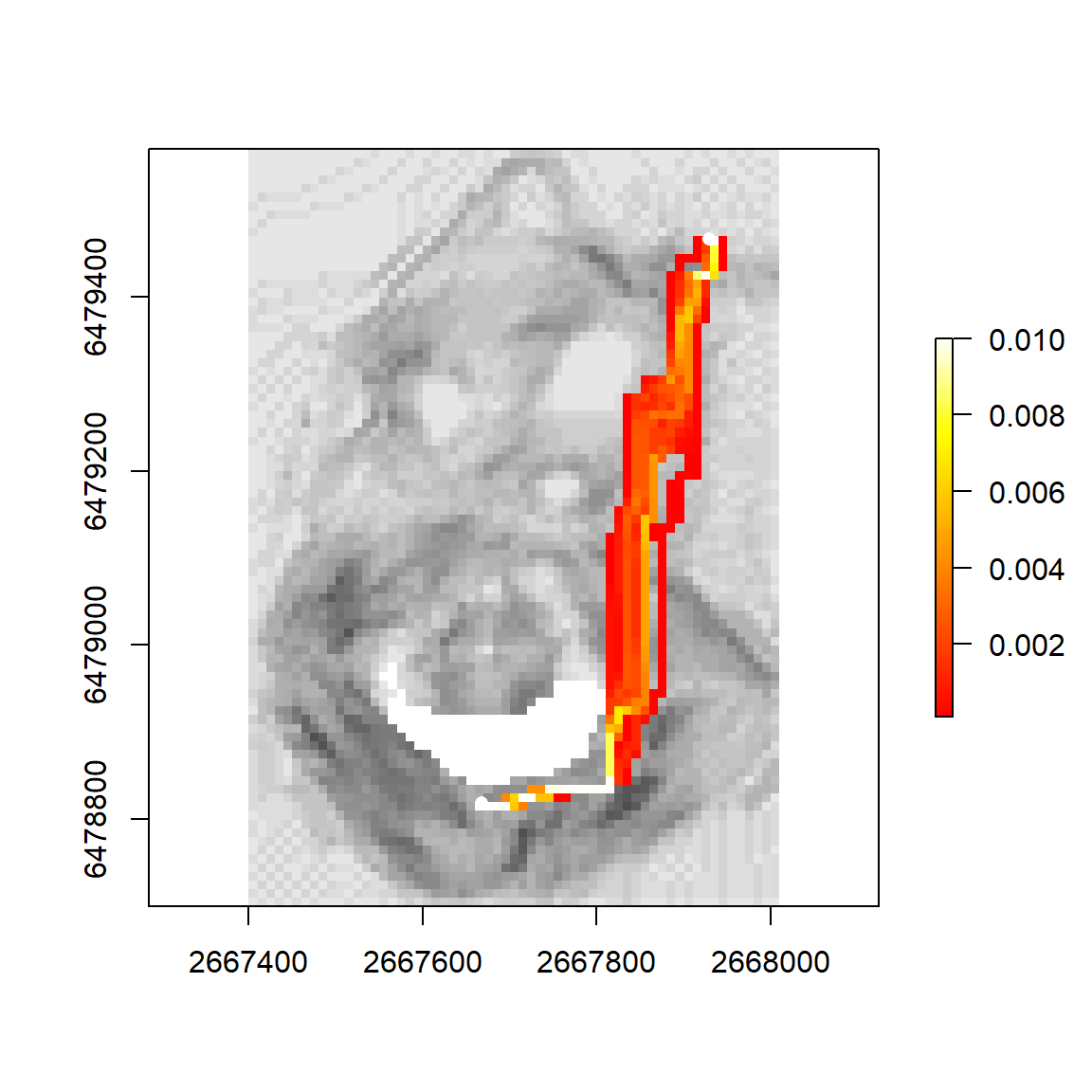plot(raster(slope_altitude_cs), legend = FALSE, col = grey.colors(100))
plot(lcps_B_A, add = T, col = heat.colors(100))
plot(A, add = T, col = "white", pch = 16)
plot(B, add = T, col = "white", pch = 16)